info@biomedres.us   +1 (720) 414-3554
One Westbrook Corporate Center, Suite 300, Westchester, IL 60154, USA

# Biomedical Journal of Scientific & Technical Research

May, 2020, Volume 27, 4, pp 20940-20942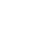Short Communication

Short Communication

# The Zeid-G Family of Distributions

Author Affiliations

Department of Biostatistics, USA

Received: May 06, 2020 | Published: May 14, 2020

Corresponding author: Clement Boateng Ampadu, Department of Biostatistics, USA

DOI: 10.26717/BJSTR.2020.27.004535

#### Abstract

In this short communication, the class of Zeid-G statistical distributions are introduced. A sub-model of this family is shown to be practically significant in fitting real-life data as well as simulated data. The researchers are asked to further develop the properties and applications of this new class.

Keywords: 1ΣαPT-Gfamilyofdistributions, Chen-G, Carbon Fibers Data

#### Introduction to the New Family

Firstly, we recall the following

Definition 1.1:  Letand ξ > 0 where α is the rate scale parameter and ξ is a vector of parameters in the baseline distribution all of whose entries are positive. A random variable Z is said to follow the (1/e)a power transform family of distributions if the Cumulative Distribution Function (CDF) is given bywhere the baseline distribution has CDF G(x;ξ ).

Proposition 1.2:  The PDF of the (1/e)a power transform family distribution is given by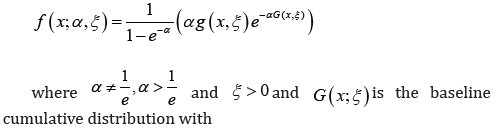probability density function g (x;ξ )

Remark 1.3: The parameter space for α have been relaxed to α ∈R,α ≠ 0. The relaxation has been employed in several papers, and for example, see 

Using this relaxation, we introduce the following, inspired by the structure of the Chen-G CDF [ 3]

Definition 1.4: A random variable Y wil bel called a Zeid random variable, if the CDF is given by Zeid-G, that is,Where the baseline distribution has CDF G(x;ξ ),ξ is a vector of parameters in the baseline CDF whose support depends on the chosen baseline CDF. α ,β ∈R, and α ,β ≠ 0

#### Practical Illustration

Assume the baseline distribution is Normal with the following CDF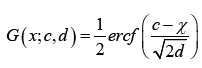where x,c∈ℝ, d > 0, and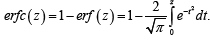. It follows from the Definition immediately above that we have the following

Proposition 2.1: The CDF of Zeid-Normal, for x∈ℝ, is given by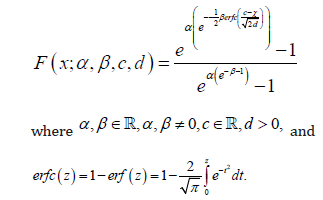Remark 2.2: We write J ∼ ZN (α ,β ,c,d ), if J is a Zeid random variable with the above
CDF
The new distribution is a good fit to real life data as well as simulated data, as illustrated below  (Figures 1 & 2)

Figure 1: The cdf of ZN (1.6156, -0.474565, 2.89578, 0.897577) (red) fitted to the empirical distribution.

Figure 2: The pdf of ZN (-1.6156, -0.474565, 2.89578, 0.897577) (blue) fitted to the histogram of 10,000 random samples of ZN (-1.6156, -0.474565, 2.89578, 0.897577).

#### Concluding Remarks

In this paper, we introduced the Zeid-G family of distributions, and showed the Zeid-Normal distribution is a good fit to real life data as well as simulated data. We hope the researchers will further develop the properties and applications of the Zeid-G class of distributions.

#### References

Short Communication

# The Zeid-G Family of Distributions

Author Affiliations

Department of Biostatistics, USA

Received: May 06, 2020 | Published: May 14, 2020

Corresponding author: Clement Boateng Ampadu, Department of Biostatistics, USA

DOI: 10.26717/BJSTR.2020.27.004535

#### Abstract

In this short communication, the class of Zeid-G statistical distributions are introduced. A sub-model of this family is shown to be practically significant in fitting real-life data as well as simulated data. The researchers are asked to further develop the properties and applications of this new class.

Keywords: 1ΣαPT-Gfamilyofdistributions, Chen-G, Carbon Fibers Data